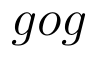Select Page

# Maths Integrals CBSE 12 Science Solutions for MCQ in English

Maths Integrals CBSE 12 Science Solutions for MCQ in English to enable students to get Solutions in a narrative video format for the specific question.

Expert Teacher provides Maths Integrals CBSE 12 Science Solutions for MCQ through Video Solutions in English language. This video solution will be useful for students to understand how to write an answer in exam in order to score more marks. This teacher uses a narrative style for a question from Integrals not only to explain the proper method of answering question, but deriving right answer too.

Please find the question below and view the Solution in a narrative video format.

Question:

Solution Video in English:

## Similar Questions from CBSE, 12th Science, Maths, Integrals

Question 1 : Evaluate :. (View Answer Video)

Question 2 : Find. (View Answer Video)

Question 3 : Write the value of :(View Answer Video)

Question 4 : Evaluate :(View Answer Video)

Question 5 : Show that :(View Answer Video)

### Relations and Functions

Question 1 : Functionsare defined respectively, by, find(View Answer Video)

Question 2 : Number of binary operations on the set {a, b} are : (View Answer Video)

Question 3 : Let * be the binary operation on N given by a * b = LCM of a and b. Find 20 * 16. (View Answer Video)

Question 4 : Functionsare defined respectively, by, find(View Answer Video)

Question 5 : If f(x) = |x| and g(x) = | 5x – 2 |. Then, fog = _____. (View Answer Video)

### Application of Derivatives

Question 1 : Find two numbers whose sum is 24 and whose product is as large as possible. (View Answer Video)

Question 2 : Equation of normal to the curve x+y=x^y where it cuts x-axis; is (View Answer Video)

Question 3 : Sand is pouring from a pipe at the rate of. The falling sand forms a cone on the ground in such a way that the height of the cone is always one-sixth of the radius of the base. How fast is the height of the sand cone increasing, when the height is 4 cm? (View Answer Video)

Question 4 : The normal at the point (1, 1) on the curveis: (View Answer Video)

Question 5 : Find two numbers whose sum is 24 and whose product is as large as possible. (View Answer Video)

### Differential Equations

Question 1 : Find the particular solution of the differential equationgiven thatwhere x = 1. (View Answer Video)

Question 2 : Write the differential equation representing the family of curves y = mx, where m is an arbitrary constant. (View Answer Video)

Question 3 : Write the degree of the differential equation :(View Answer Video)

Question 4 : Write the degree of the differential equation(View Answer Video)

Question 5 : Solve the differential(View Answer Video)